## Saturday, January 31, 2015

### Right Circular Cylinder Problems, 12

Category: Solid Geometry

"Published in Newark, California, USA"

A wedge ABCDEF (see figure) is cut from a right circular cylindrical block of altitude 10 in. and radius 4 in. The dihedral angle of the wedge is 30°. Calculate its volume and total surface.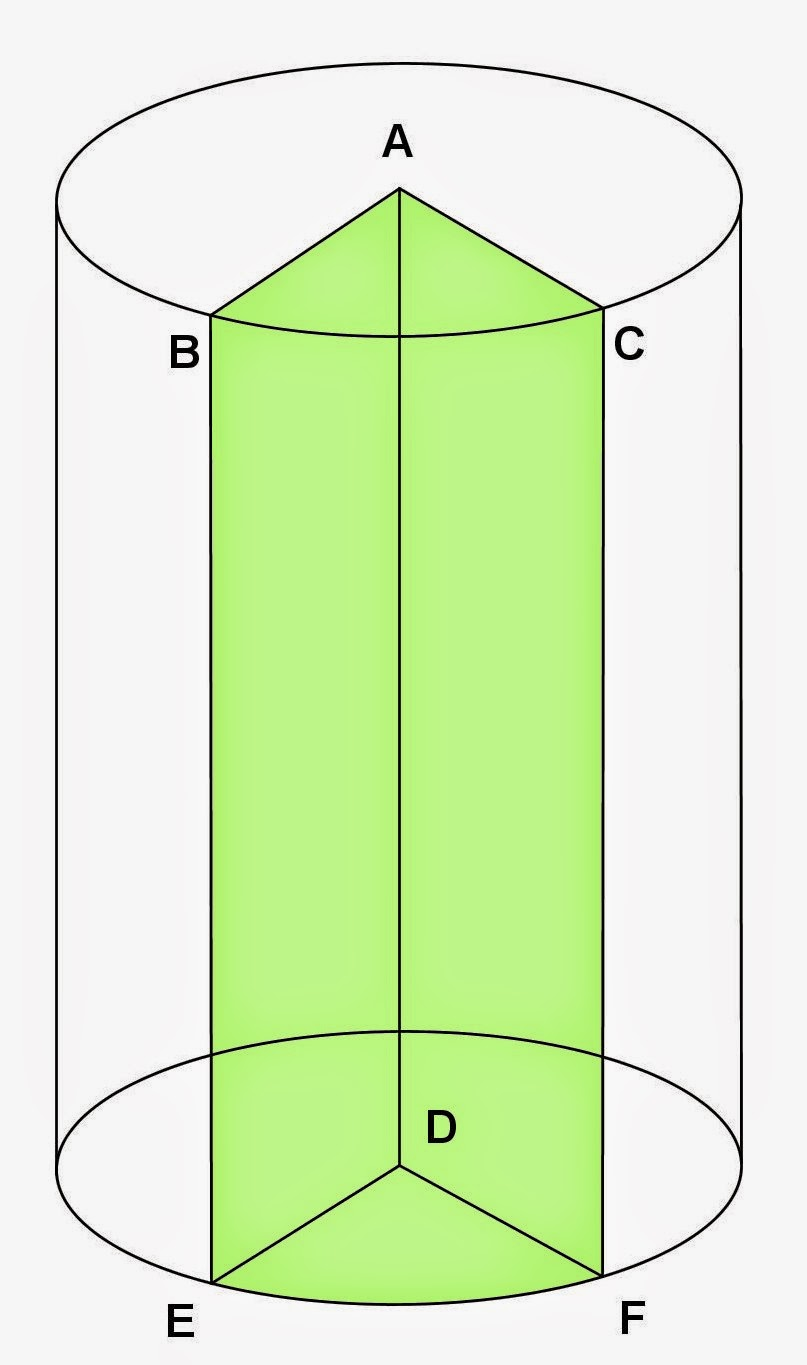Photo by Math Principles in Everyday Life

Solution:

To analyze more the problem, it is better to label further the given figure as follows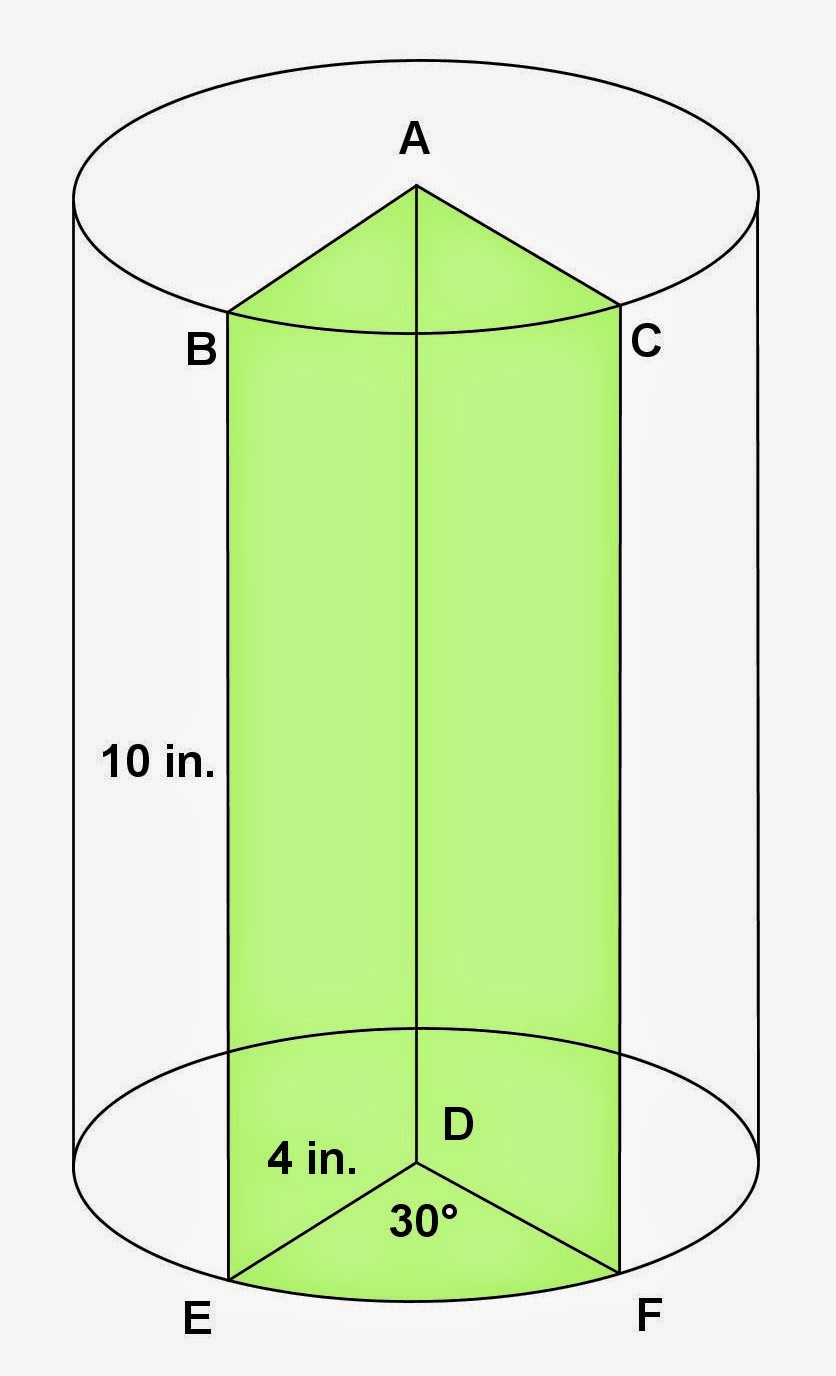Photo by Math Principles in Everyday Life

In this problem, we will use the given angle in solving for the length of an arc as well as the area of a circular sector. The given angle should be expressed in radians because it is a unitless value.

The area of a circular sector DEF is

Therefore, the volume of wedge ABCDEF is

The length of arc EF is

Therefore, the total surface area of wedge ABCDEF is

## Friday, January 30, 2015

### RIght Circular Cylinder Problems, 11

Category: Solid Geometry, Physics

"Published in Newark, California, USA"

When a body is immersed in water in a right circular cylinder 60 cm. in diameter, the level of the water rises 40 cm. What is the volume of the body?

Solution:

To illustrate the problem, it is better to draw the figure as follows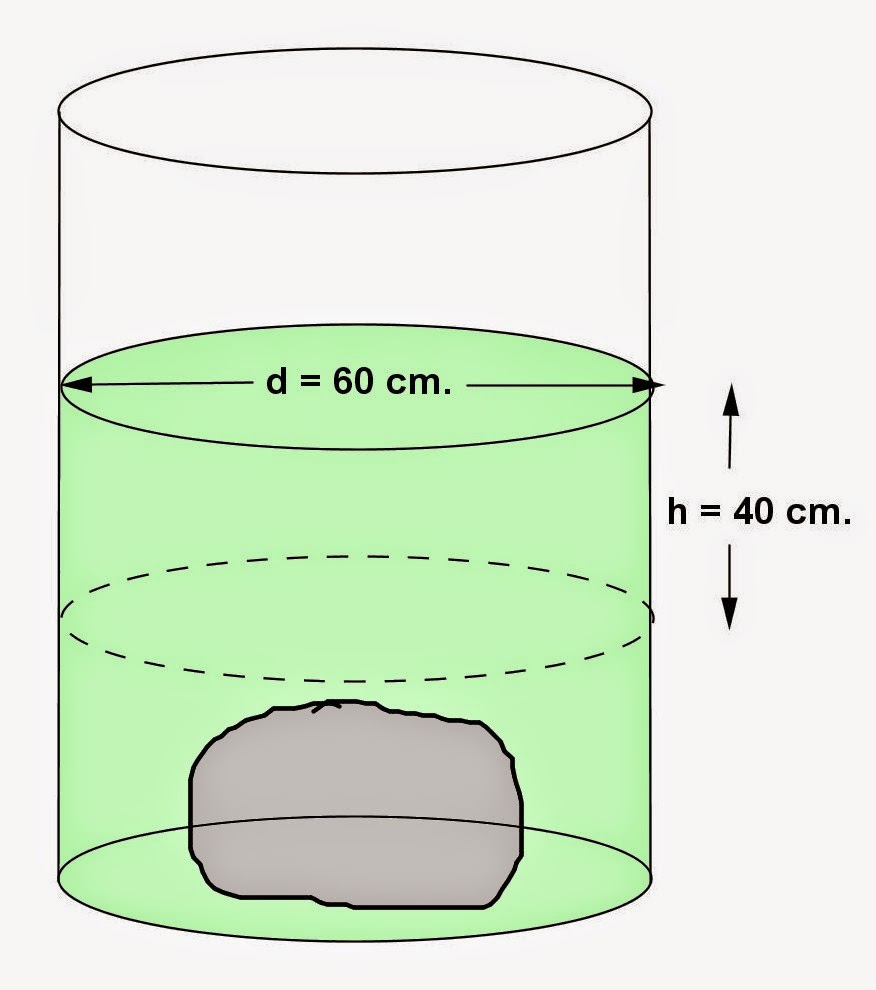Photo by Math Principles in Everyday Life

If you have an object with irregular shape, let's say a stone, for example, then it is difficult to get its volume. In this case, the only way to get the volume of an object is by immersion method. You will need a right circular cylinder with water and measure its height. Next, immerse an object in water in a right circular cylinder and measure its height. The difference of their height will be used to calculate the change of the volume of water which is equal to the volume of an object.

Since the change of the level of water in a right circular cylinder is given in the problem, therefore, the volume of an object is

## Thursday, January 29, 2015

### Right Circular Cylinder Problems, 10

Category: Solid Geometry

"Published in Vacaville, California, USA"

A cylindrical standpipe is to contain 343,000 gal. of water. If its height is to be twice its diameter, what must be its dimensions?

Solution:

To illustrate the problem, it is better to draw the figure as followsPhoto by Math Principles in Everyday Life

Since the given volume of water is in gallons, then we have to convert it first into cubic feet as follows

Hence, the diameter of a cylindrical standpipe is

and its height is

Therefore, the dimensions of a cylindrical standpipe are d = 30.791030 ft. and h = 61.58206 ft.

## Wednesday, January 28, 2015

### Right Circular Cylinder Problems, 9

Category: Solid Geometry

"Published in Newark, California, USA"

A log with a circular cross section is 15 ft. long and 27 in. in diameter at the smaller end. Find the dimensions of the largest piece of timber of length 15 ft. with a uniform square cross section , that can be cut from the log.

Solution:

To illustrate the problem, it is better to draw the figure as followsPhoto by Math Principles in Everyday Life

In this problem, we will cut the log in order to get the largest piece of timber of the same length which is 15 ft. and the cross section is a square. Now, let's consider the cross section of a log after it is cut as follows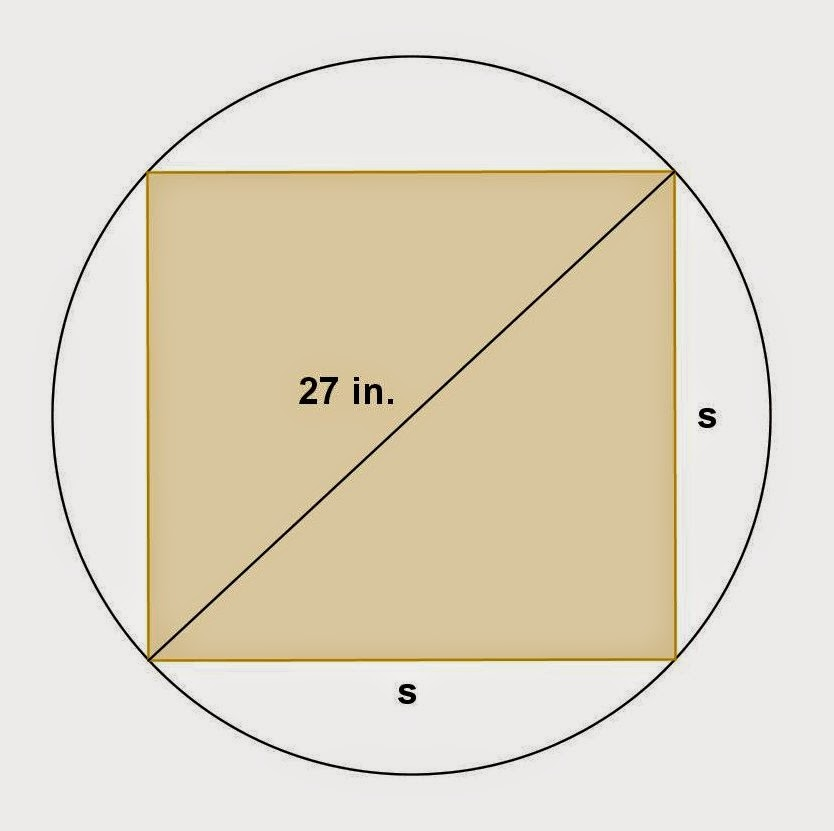Photo by Math Principles in Everyday Life

The diameter of a log is the hypotenuse of a cross section which is a square that passes through its center. By Pythagorean Theorem, the length of the sides of a square is

or

Therefore, the dimensions of a largest timber that can be cut from the log is## Tuesday, January 27, 2015

### Right Circular Cylinder Problems, 8

Category: Solid Geometry

"Published in Newark, California, USA"

A circular oak table top is 4 ft. in diameter and ¾ in. thick. How heavy is it if oak weighs 47 lb. per cu. ft.?

Solution:

To illustrate the problem, it is better to show the figure as follows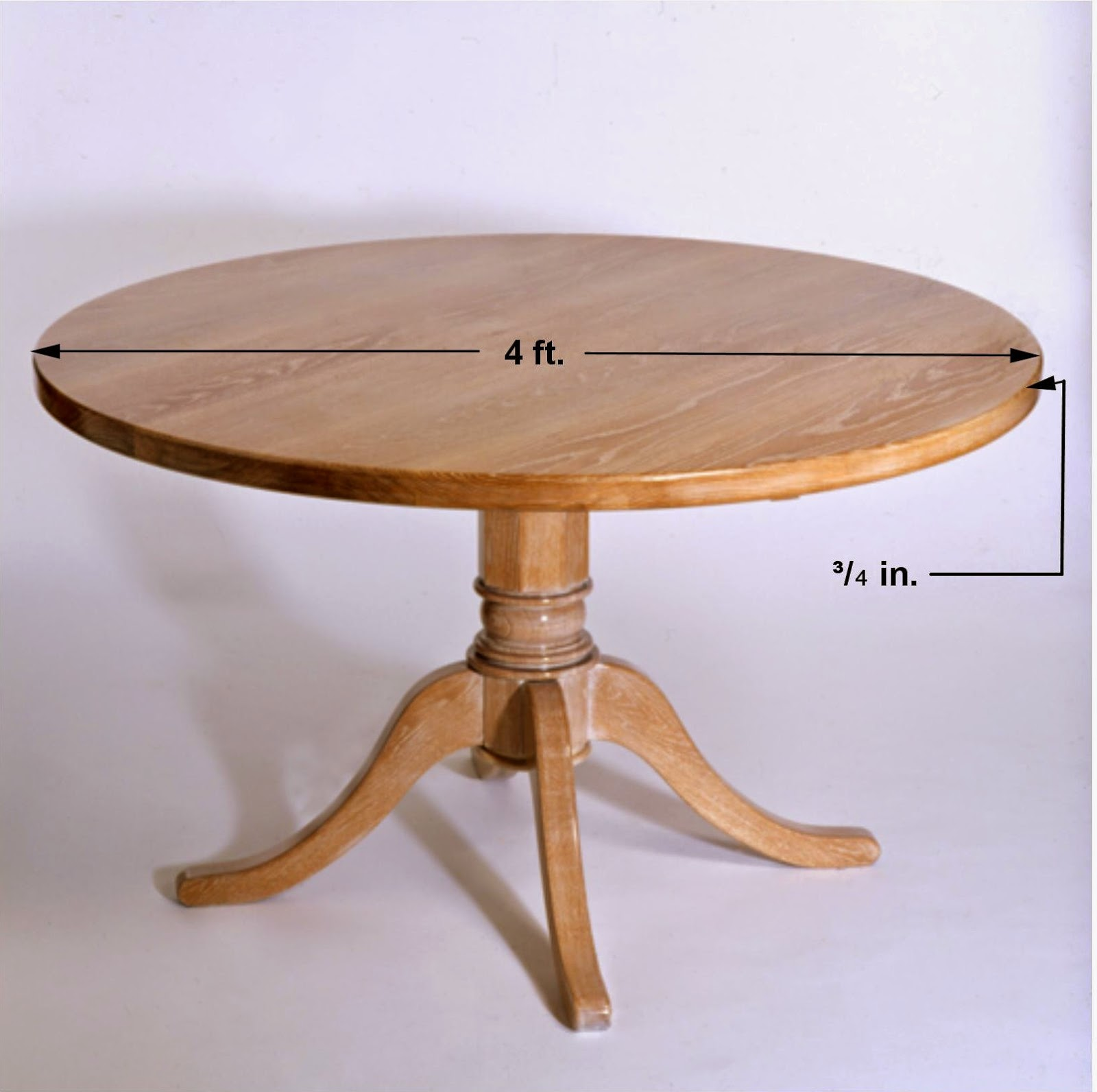Photo by Math Principles in Everyday Life

The volume of a circular oak table top which is the volume of a right circular cylinder is

Therefore, the weight of a circular oak table top is